# Syntax

What is syntax and how does it differ, from say, semantics? What are the different ways that we can define a syntax? What is the difference between concrete and abstract syntax? And why do programming languages tend to use context-free grammars for their syntax?

## Motivation

A language gives us a way structure our thoughts. Utterances in a language (called programs in a programming language) have a kind of internal structure, for example: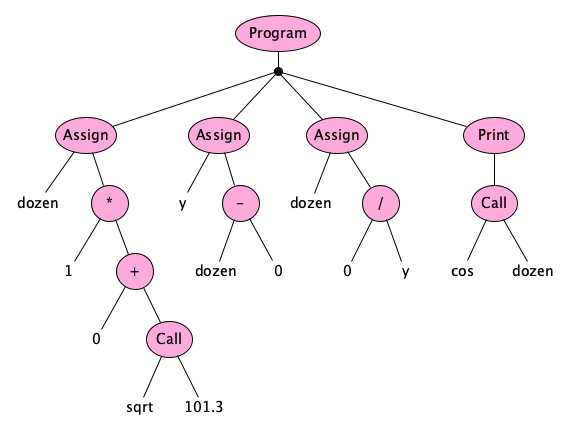We generally capture this structure as a sequence of symbols; for example, the structure above might appear as:

dozen = 1 * (0 + sqrt(101.3));
y = dozen - 0;   // TADA 🥑
dozen = 0 / y;
print cos(dozen);//


or even:

MOVE PRODUCT OF 1 AND (SUM OF 0 AND SQRT OF 101.3) INTO DOZEN.
MOVE DIFFERENCE OF DOZEN AND 0 INTO Y.
MOVE QUOTIENT OF 0 AND Y INTO DOZEN.
PRINT COSINE OF DOZEN.
COMMENT


or even:

(program
(assign dozen (* 1 (+ 0 (call sqrt 101.3))))
(assign y (- dozen 0))            ; TADA 🥑
(assign dozen (/ 0 y))
(print (call cos dozen))          ;


Of course, we don’t have to use strings! Visual languages do exist: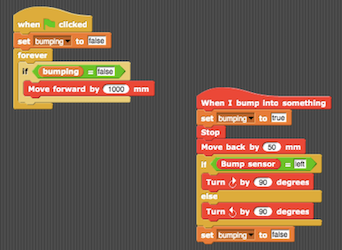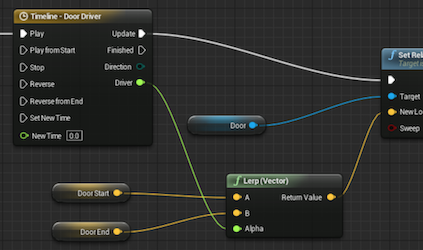Text vs. Pictures

Text is more dense than pictures, and more directly machine readable at times (no computer vision machine learning necessary). Even if your language was expressed with pictures, there will be an underlying byte-array, or string, representation for storage and transmission.

So our focus from now on will be on text.

Exercise: Text is by no means always better than pictures! Please watch Bret Victor’s The Future of Programming for thoughts on programming visually.

If you were a language designer, you’d deal with questions such as:

• Is code expressed in terms of blocks? Visual graphs? Text?
• If text, which characters are allowed? Is whitespace significant? Is case significant?
• Is there a lot of punctuation? Symbols? Or is it mostly words? If words, what natural language are you biased toward?
• How do we do comments? Pragmas? Directives?
• Do we express structure with indentation, curly-braces, terminating-end, or nested parentheses?
• Should operations have a functional (length(a)) or message-oriented (a.length) feel?
• What kind of shorthands and idioms should be made available?
• How do program units map to files or other physical containers?

## Definition

The syntax of a programming language is the set of rules that define which arrangements of symbols comprise structurally legal programs—and for each structurally legal program, what its structure actually is.

Syntactic rules should be able to tell us that these sentences are not well-formed in English:

• telescope a they the With hit saw.
• yqvfe.,--)AO7%;;;;;;;; ; !dogs

but that these are:

• They hit the telescope with a saw.
• They saw the hit with a telescope.

and so is this famous one, due to Chomsky:

• Colorless green ideas sleep furiously.

## Learning by Doing

Let’s learn about syntax by going through the process of designing a syntax for a small language.

We’ll assume the preliminary phases of language design—identifying the purpose (education), scope (simple arithmetic computation), audience (students), and name (Astro) of our language—have been done, and now it is time for the technical work to begin.

We first sketch a program to illustrate the syntactic features we want:

// A simple program in Astro

radius = 55.2 * (-cos(2.8E-20) + 89) % 21;    // assignment statement
the_area = π * radius ** 2;                   // a built-in identifier
print hypot(2.28, 3 - radius) / the_area;     // print statement


Next, we put our ideas into words. A first pass: “Programs are structured as a sequence of one or more statements, each of which is an assignment or print statement, with expressions formed with numbers, variables, function calls, and the usual arithmetic operators, which can have parentheses when needed. Comments look like the slash-slash comments of C-like languages.”

Natural language isn’t super precise, so as we sometimes do in design, we’ll next turn thoughts into pictures.

A program is a sequence of one or more statements:

Program

There will be two kinds of statements: assignments and print statements:

Statement

Assignments and print statements look like this:

Assignment
PrintStmt

An identifier is the computer science word for a name of something you define in your code, like a variable, constant, function, type, parameter, or similar thing. A keyword is a special word in your language, like let or if or while—these words generally do not name things—they are just used to structure the code. If a keyword is not allowed to be an identifier, we call it a reserved word. Astro has one reserved word: print.

Identifiers in Astro begin with a letter, and can have letters, digits, and underscores (examples: x, last_attempt, p1, p2, overTheLimit, bot8675309_jEnNy). We will call letters, digits, and underscores identifier characters (idchars). But notice that print is not allowed to be an identifier:

id
idchar

How is the reserved word print specified? We have to be careful. It’s not just the five letters p, r, i, n, and t. If it were then the program:

    printy;


would be legal! It would be the five characters spelling print followed by a legal expression, namely the identifer $y$. We want the word print to not bleed into any following characters that might be part of an expression. In other words, print must not be immediately followed by an identifier character:

print
Exercise: Make sure you understand how this definition ensures that “printy” is an identifier and not print followed by the identifier y.

A statement performs an action (with a side-effect), but expressions represent the computation of a value. Expressions can be numbers, identifiers (representing variables), function calls, or can be made up of operators applied to other expressions. We can put parentheses around any expression. This will do the trick (but spoiler alert: we will have to change it later):

Exp
Call
Exercise: Explain the difference between expressions and statements to a friend. Make yourself a sacred vow never to mix the terms up. Precise language is a good thing. Sometimes we slip up, but still, let’s try not to say things like “plus statement.”

We mentioned numerals here, so let’s define these. We’ll keep things in decimal only (no worries about hex or binary), and use the times-ten-to-the notation from popular programming languages:

num

What about letters and digits? Well digits are pretty easy:

digit

For letters, well, there are tens of thousands of these in Unicode, so we’ll just say that one is “built-in.”

## Lexical and Phrase Syntax

Did you notice we used two kinds of nodes in our syntax diagrams? Ovals are for nodes that internally cannot have spaces in them (lexical nodes, or tokens); rectangles are used for constructs whose components can have spaces between them (phrase nodes, or phrases). The special category called space defines what can separate tokens within phrases.

space

The above are the “spaces” that separate constructs in phrases. They are the space character, the tab character, the carriage return character, the linefeed character (the linefeed (U+000A) is pretty much universally accepted as the “newline”), and comments. Comments function as spaces, too! For comments, we chose to start with // and go to the end of the current line.

We also followed an accepted convention to start the names of lexical nodes with lowercase letters and capitalize the names of phrase nodes.

Another convention we will follow for programming language syntax is to take the following as predefined:

• letter, for any Unicode letter
• digit, for "0".."9"
• alnum, for letter | digit
• upper, for any Unicode uppercase letter
• lower, for any Unicode lowercase letter
• hexDigit, for digit | "a".."f" | "A".."F"
• any, for any Unicode character at all

and also allow these conveniences:

• \u{ and }, delimit a code point in hex and stands for the unicode character with that code point
• \n, for the LF character (same as \u{a})
• \r, for the CR character (same as \u{d})
• \t, for the tab character (same as \u{9})
• \", for the quote character (same as \u{22})
• \', for the apostrophe character (same as \u{27})
• \\, for the backslash character itself (same as \u{5c})

Note that any can be defined as \u{0}..\u{10ffff}.

Remember

The term space, though it looks like a lexical node, is something meta; it is treated specially.

Let’s spend some more time on the philosophical significance of the separation of lexical and phrase syntax.

The lexical syntax, with the exception of the special rule for space, show us how to combine characters into words and punctuation which we’ll call tokens. The phrase syntax show us how to combine words and symbols into phrases (where the words and symbols can, if you wish, be surrounded by spacing).

Exercise: Meditate on how profound this is. Have you seen anything like this before? Hint: Are you reading this page in English?

Now if we wanted to recognize whether a string is “in” a language, we can figure this out in two phases. The first phases uses the lexical rules to tokenize a stream of characters into a stream of tokens, and the second phase uses the phrase rules to parse the token stream into the parse tree. Here’s an example. The source string:

  print( // ⿇🌿
420 );


is made up of these characters:

SPACE SPACE LATIN SMALL LETTER P LATIN SMALL LETTER R LATIN SMALL LETTER I LATIN SMALL LETTER N LATIN SMALL LETTER T LEFT PARENTHESIS TAB SOLIDUS SOLIDUS SPACE KANGXI RADICAL HEMP HERB LINEFEED DIGIT FOUR DIGIT TWO DIGIT ZERO SPACE RIGHT PARENTHESIS SEMICOLON

When we follow the lexical rules to this character stream, skipping the spaces (and comments), we get the token stream:

print ( num(420) ) ;

Next, we follow the phrase rules allows us to uncover the parse tree: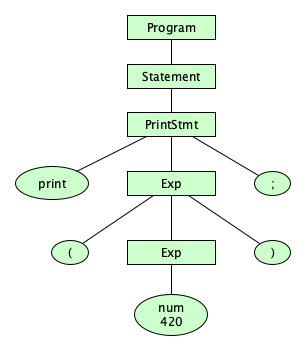A very important thing to note: The frontier of the parse tree is the token stream.

Exercise: Repeat this phrase to yourself five times: The frontier of the parse tree is the token stream.
The parse tree ends at tokens, not characters

Please take the time to consider how silly it would be if the parse tree expanded all of the lexical rules down to characters. Having the parse tree stop at tokens is a good example of what we would call “breaking a complex problem down into simpler parts.”

## Dealing With Ambiguity

How are we doing so far? We are able to distinguish well-structured Astro programs from all other Unicode strings. But there are some things we haven’t dealt with yet. For one, we have some strings with multiple structural forms. For example, the phrase 9-3*7 can be parsed in two ways: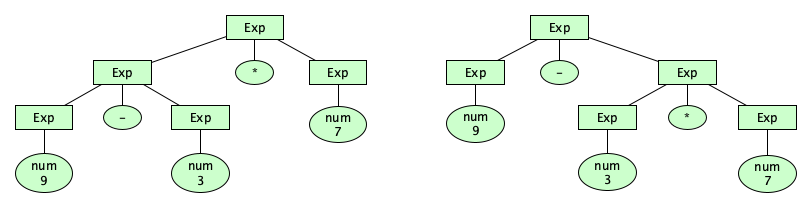Having more than one parse tree for a given input string means that our syntax description is ambiguous. It’s possible to handle this particular kind of ambiguity in the syntax. Here’s how.

### Precedence

We can create rules that force certain operators to be applied before others; that is, the precedence of operators can be enforced in our syntax definition. To do so, we define additional syntactic categories. We say:

• An expression is a sequence of one or more terms, separated by additive operators.
• A term is a sequence of one or more factors, separated by multiplicative operators.
• A factor is made up of primaries, separated by exponentiation operators, or a factor can just be a negated primary.
• A primary is the most basic expression possible: either a simple identfier, a numeral, a call, or a parenthesized expression. Parentheses allow unlimited nesting.

We can write:

Exp
Term
Factor
Primary

Great! Now there is one and only one parse tree for that previously problematic expression: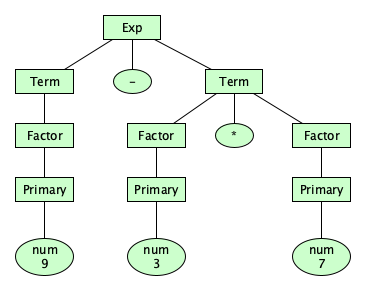Note that the new syntax has forced the binary operators into a precedence hierarchy!

• + and - have the lowest precedence.
• *, /, and / have the next higher precedence.
• ** has the highest precedence.

Of course, you can think of parenthesized expressions as being done before anything else, though we don’t usually think of these as operators.

### Associativity

Wait, we are not done with structuring our operators just yet. The way things stand now, the parse tree for 3-8-5 looks pretty flat: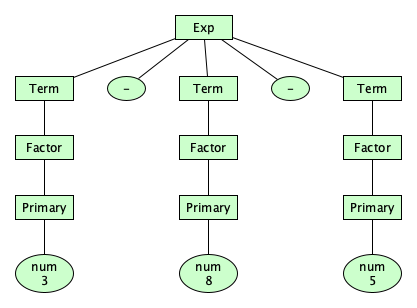It doesn’t suggest whether we mean to compute (3-8)-5 (= -10) or 3-(8-5) (= 0). As language designers, we should make the choice of left-associativity or right-associativity or even non-associativity for each operator. There is a way to do this. Let’s make the additive and multiplicative operators left associative and the exponentiation operator right associative:

Exp
Term
Factor

How the heck does this work? Study these parse trees, and hopefully the insight will come to you! (Hint: remember the syntax is designed to force the tree to “come out” a certain way.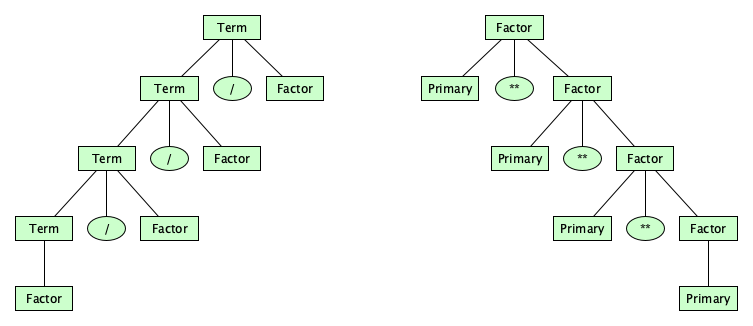Exercise: Study this until you understand it well.

## Parsing

We’ve made syntax diagrams to describe all the legal programs in the language, but how to we follow the diagrams to “match” or “parse” a candidate program? That is, given a program (a candidate string), how, exactly, do we figure out how it is matched by, or generated by, the syntax diagrams? How do we parse?

There have been hundreds of books and thousands of papers on the topic of parsing over the years. Here we just need to highlight a few things.

### Hand-Crafted Parsers

Because a parser is just a program that turns source code into a parse tree, you can just write on by hand. The most common kind of hand-crafted parser uses the technique of recursive descent, which you can read about at Wikipedia. You kind of walk through each of the syntax diagrams.

CLASSWORK
I’ve written a recursive descent parser as part of an Astro compiler. We’ll take a very brief look at it in class.

### Parser Generators

A recursive descent parser generally requires a highly restricted syntax, one that is line with a class of languages known as the $LL(k)$ languages. These languages are a proper subset of the $LR(k)$ languages (also known as the deterministic context-free languages), which are used in popular parser generators such as YACC, Bison, and ANTLR, among others. While these systems are quite popular and famous, we won’t be covering them here. Do read about them, though.

We will see $LL(k)$ and $LR(k)$ later in the course, though.

### Analytic Grammars

Another approach is to use a specialized kind of grammar designed specifically for parsing. So instead of using traditional generative grammars, covered previously in our notes on Language Theory (which specify a language by giving a set of rules to generate all and only those strings in a language), we can use an analytic grammar, in which the rules specify how to match a candidate string.

The biggest difference between generative and analytic grammars is in the handling of alternatives. In a generative grammar $G$, $L(G)$ is contains all strings that can be generated by applying rules whenever possible. For example, the rule $S \rightarrow A\,|\,B$ means you can replace an $S$ with an $A$ or a $B$, so you can generate both. So the grammar:

$s \longrightarrow \texttt{"a"}\;|\;\texttt{"ab"}$

describes the language $\{ a, ab\}$.

In an analytic grammar, the rules are deterministic; you don’t get to pick one or the other. So $A\,/\,B$ means: “Try to match $A$. If you can, the rule matches and you are done. Otherwise (and only otherwise), try to match $B\,$”. So:

$s \longleftarrow \texttt{"a"}\;/\;\texttt{"ab"}$

recognizes the language $\{ a \}$, but:

$s \longleftarrow \texttt{"ab"}\;/\;\texttt{"a"}$

recognizes the language $\{ a, ab \}$.

Note the tradeoff here. Determinism means there’s never ambiguity (a string can only parse one way, if it can at all), which is great! But the ordering of the rules matters, so you have to be careful to do what you want. Here’s another illustration. If we wanted an analytic grammar for Astro, the rule:

$\mathit{Primary} \longleftarrow \mathit{num}\;/\;\mathit{id}\;/\;\mathit{Call}\;/\;\ldots$

would be wrong, as it will never match a call!

Exercise: Why, exactly, must the Call alternative precede the id alternative?
CLASSWORK
This might be deep, so we’ll try a few more examples.

Unlike generative grammars, analytic grammars feature special lookahead operators, including the negative lookahead operator (~) and the positive lookahead operator (&). This enables the definition of non-context-free languages, despite having only one symbol on the left-hand side! Here’s an analytic grammar for the non-context-free language $\{ a^nb^nc^n \,\mid\, n \geq 0 \}$:

$\begin{array}{lcl} \mathit{s} &\longleftarrow& \texttt{&} \; (\mathit{x}\texttt{ "c"}) \; \texttt{"a"}^+\;\mathit{y} \\ \mathit{x} &\longleftarrow& \texttt{"a"} \; \mathit{x}? \; \texttt{"b"} \\ \mathit{y} &\longleftarrow& \texttt{"b"} \; \mathit{y}? \; \texttt{"c"} \end{array}$

### Parsing Expression Grammars (PEGs)

There are various formalisms of analytic grammars (Wikipedia identifies several); the most popular is the Parsing Expression Grammar (PEG). To read a PEG description, pronounce the operators as follows:

• A B: match A then match B
• A / B: match A. If it matched, success. Otherwise, match B
• A?: match A zero or one times
• A*: match A zero or more times
• A+: match A one or more times
• &A: match A without consuming it (but it must match)
• ~A: match A without consuming it. If A matches then ~A fails. If A fails ot match, then ~A succeeds
• end: matches the end of the string
• Quoted text: successively match each symbol exactly

Note how PEGs use / instead of |, perhaps to emphasize the deterministic nature of matching.

Strictly speaking, it should be impossible in a PEG to have left-recursive rules like:

$\mathit{Exp} \; \longleftarrow \mathit{Exp} \; \texttt{"+"} \; \mathit{Term} \;\;/\;\; \mathit{Term}$

given that “in order to match an Exp, you much first try to match an Exp.” It’s sounds circular. In fact it is, and traditionally, PEG implementations suggested using the rule:

$\mathit{Exp} \; \longleftarrow \mathit{Term} \; (\texttt{"+"} \; \mathit{Term})^*$

instead. However, it turns out a proper parsing technique (using a packrat parser) can deal with it! It's not easy, but you can read the original paper by Alex Warth et al or this nice and detailed article by Guido van Rossum to see how it’s done.

Analytic grammars:

• Are designed for parsing (recognition) rather than generation of languages.
• Are written with only a single variable on the left hand side of each rule.
• Use $\longleftarrow$ rather than $\longrightarrow$ to define rules.
• Execute alternatives left-to-right and once you get a match, you keep it, even if it leads to a dead end.
• Have powerful lookahead operators, thanks to deterministic execution.
• Can handle left-recursive rules.

Try this exercise before moving on:

Exercise: Show that the following generative grammar for assignment statements and if-then-else statements in a programming language:
    S ⟶ "a" | "i" "x" "t" S ("e" S)?
is ambiguous, but that the ambiguity can be resolved by rewriting as a PEG:
    S ⟵ "a" / "i" "x" "t" S "e" S / "i" "x" "t" S
How, exactly, is the ambiguity resolved? (Historical note: this is the dangling else problem, a classic in parsing theory.)

Now let’s put everything together to come up with a Parsing Expression Grammar for Astro, that (1) properly separates lexical and phrase rules, (2) deals properly with precedence and associativity, (3) embraces left-recursion, and (4) correctly handles reserved words.

$\begin{array}{lcl} \mathit{Program} &\longleftarrow& \mathit{Statement}^+ \\ \mathit{Statement} &\longleftarrow& \mathit{Assignment} \;/\; \mathit{PrintStmt} \\ \mathit{Assignment} &\longleftarrow& \mathit{id}\;\texttt{"="}\;\mathit{Exp}\;\texttt{";"} \\ \mathit{PrintStmt} &\longleftarrow& \mathit{print}\;\mathit{Exp}\;\texttt{";"} \\ \mathit{Exp} &\longleftarrow& \mathit{Exp} \;(\texttt{"+"}\,|\,\texttt{"–"})\; \mathit{Term} \;/\; \mathit{Term} \\ \mathit{Term} &\longleftarrow& \mathit{Term} \;(\texttt{"*"}\,|\,\texttt{"/"}\,|\,\texttt{"%"})\; \mathit{Factor} \;/\; \mathit{Factor} \\ \mathit{Factor} &\longleftarrow& \mathit{Primary}\;(\texttt{"**"}\;\mathit{Factor})? \;/\; \texttt{"–"} \; \mathit{Primary} \\ \mathit{Primary} &\longleftarrow& \mathit{num} \;/\; \mathit{Call} \;/\; \mathit{id} \;/\; \texttt{"("} \; \mathit{Exp} \; \texttt{")"} \\ \mathit{Call} &\longleftarrow& \mathit{id}\;\texttt{"("}\;(\mathit{Exp}\;(\texttt{","}\;\mathit{Exp})^*)?\;\texttt{")"} \\ \mathit{num} & \longleftarrow & \mathit{digit}^+ \; (\texttt{"."} \; \mathit{digit}^+)? \; ((\texttt{"E"} | \texttt{"e"}) \; (\texttt{"+"} | \texttt{"–"})? \;\mathit{digit}^+)? \\ \mathit{print} &\longleftarrow& \texttt{"print"}\;\neg\mathit{idchar}\\ \mathit{id} &\longleftarrow& \neg\mathit{print}\;\mathit{letter}\;\mathit{idchar}^*\\ \mathit{idchar} &\longleftarrow& \mathit{letter}\,|\,\mathit{digit}\,|\,\texttt{"_"}\\ \mathit{space}&\longleftarrow& \texttt{" "}\,|\,\texttt{"\t"}\,|\,\texttt{"\r"}\,|\,\texttt{"\n"}\,|\,\texttt{"//"}\;(\neg\texttt{"\\n"} \; \mathit{any})^* \end{array}$

Lexical and Phrase Variables

Ohm also adopts the convention of starting the names of lexical variables with a lowercase letter and phrase variables with an uppercase letter.

### Ohm

If you want to build your own interpreters and compilers, Ohm is a powerful language library that comes with its own grammar notation! Ohm grammars are analytic grammars. They are very similar to Parsing Expression Grammars but have a few differences that make it useful to write large, maintainable, and sophisticated language processors.

We will be studying Ohm in great detail soon. For now, here is the Ohm grammar for Astro, which we’ll explore briefly in class:

Astro {
Program     = Statement+
Statement   = id "=" Exp ";"                         --assignment
| print Exp ";"                          --print
Exp         = Exp ("+" | "-") Term                   --binary
| Term
Term        = Term ("*" | "/" | "%") Factor          --binary
| Factor
Factor      = Primary "**" Factor                    --binary
| "-" Primary                            --negation
| Primary
Primary     = id "(" ListOf<Exp, ","> ")"            --call
| numeral                                --num
| id                                     --id
| "(" Exp ")"                            --parens

numeral     = digit+ ("." digit+)? (("E" | "e") ("+" | "-")? digit+)?
print       = "print" ~idchar
idchar      = letter | digit | "_"
id          = ~print letter idchar*
space      += "//" (~"\n" any)*                      --comment
}


By the way, there is a killer live editor for Ohm grammars that facilitates language design and grammar testing. It makes prototyping and experimenting so easy (and fun)!

## The Problem of Context

Question: Does the grammar we have defined above for Astro capture the following (desirable) rule:

“You can only use an identifier if it has been previously declared.”

Exercise: Show that it does not. Hint: Is print(x); a legal program according to the grammar?
Exercise: Show how to capture the rule in a grammar. Hint: Do not be frustrated if you cannot.

Wait, this is an incredibly hard thing to do! Enforcing this rule requires knowledge of context. That is, the syntactic rule for producing expressions such as calls and arithmetic operations would need to somehow know which identifiers were previously declared. How can the grammar capture that?

It turns out that the designers of real programming languages actually leave enforcement of contextual rules out of the grammar! In fact, while the official grammar for Java will not derive this program:

class A {2 / == {{;


and report a syntax error, a Java compiler will say that this program:

class A {int x = y;}


is structurally well formed according to the official syntax of the Java language! The compilation unit consists of a type declaration that is a class declaration whose body consists of a field declaration with a type, a name, and an initializing expression which is an identifier. But we know this program is not legal, since the identifier y has not been declared. What’s going on? The same goes for this C program, which has multiple problems despite being “structurally well-formed” according to the C grammar:

/*
* This program is derivable by the official grammar for C.
*/
int main() {
f(x)->p = sqrt(3.3);
return "dog";
}


So why do these language grammars allow this nonsense? The short answer is, it’s really really really really really really really really hard and really really really really really really really really ugly to define contextual rules grammatically! Capturing the “all identifiers must be declared before use” constraint would require that a grammar rule for expressions not just generate any identifier, but only certain identifiers known from some outside context. Context! In general, capturing context in a grammar leads to grammars that:

• are incredibly difficult for humans to figure out,
• are nearly impossible for humans to read (they’re ridiculously long and incredibly weird),
• look nothing at all like the way we would check these rules in a compiler,
• give rise to trees that are weird since expansion of nodes is based on sibling nodes, not just the parent, which makes semantics hard to define,
• and
• horribly expensive even for a computer to parse.

Try the following exercise if you’re not convinced:

Exercise: Write grammars for:
• $\{ a^nb^nc^n \;\mid\; n \geq 0 \}$
• $\{ a^{2^n} \;\mid\; n \geq 0 \}$
• $\{ ww \;\mid\; w \in \{a,b\}* \}$
Hint 1: Grammars for these languages will have to have at least some rules with multiple symbols on the left hand side. That is because there exist proofs (not shown here) that these three languages are not context-free.

Hint 2: Having trouble? Answers can be found on my notes on Language Theory.
Exercise: Do some research to see if there are any known lower complexity bounds on the parsing of context-sensitive grammars.

Sometimes there are constraints that we can (theoretically) capture in a context-free syntax, but that we might not want to. Try the following two exercises:

Exercise: Write a syntax rule for nonnegative integers less than $128$.
Exercise: Write a syntax rule for nonnegative integers less than $2^{63}$. Is this busy work?

### Defining Contextual Constraints

If you think about it, so many constraints require context. Here’s a small sampling from various languages:

• Identifiers must not be redeclared within a scope
• Identifiers may not be used in a place where they might not have been initialized
• Types of all subexpressions must be consistent in an expression
• The correct number of arguments must appear in a call
• Access modifiers (private, protected, public) must be honored
• All execution paths through a function must end in a return
• All possible arms of a match or switch or discriminated union must be covered
• All abstract methods must be implemented or declared abstract
• All declared local variables must be used
• All private methods in a class must be used
• ...and so many more

For our running example language, Astro, our constraints are:

1. The following identifiers are built-in:
• π, a number
• sqrt, a function of exactly one argument
• sin, a function of exactly one argument
• cos, a function of exactly one argument
• hypot, a function of exactly two arguments
2. An identifier cannot be used in an expression unless it is one of the built-in identifiers or has been previously assigned to.
3. All function calls must accept the proper number of arguments.
4. The built-in identifiers cannot be assigned to.
5. Identifiers declared as functions can only be called, not used in any other context. Identifiers declared as numbers cannot be called.

That’s informal prose. Can we capture the rules in a formal notations? Well, context sensitive grammars are too hard to write, too hard to understand, and too hard to parse, so we need other options. There are at least two:

• Use a completely new kind of grammatical formalism. Some of these do exist, including Attribute Grammars and Two-Level Grammars.
• Push contextual constraints into the world of semantics. Let the syntax be happy with undeclared identifiers appearing in expressions (as long as the expressions are otherwise well formed). With this approach, we say that using undeclared identifiers is ”meaningless,” you know, a semantic problem. And because the meaning (ahem, semantics) is computed before the program is run, we would call constraint violations static semantic errors.

Which take do you prefer? Slonneger and Kurtz take the former approach, writing:

The classification of such an error entails some controversy. Language implementers, such as compiler writers, say that such an infraction belongs to the static semantics of a language since it involves the meaning of symbols and can be determined statically, which means solely derived from the text of the program. We argue that static errors belong to the syntax, not the semantics, of a language. Consider a program in which we declare a constant:
    const c = 5;
In the context of this declaration, the following assignment commands are erroneous for essentially the same reason: It makes no sense to assign an expression value to a constant.
    5 := 66;
c := 66;
The error in the first command can be determined based on the context-free grammar (BNF) of the language, but the second is normally recognized as part of checking the context constraints. Our view is that both errors involve the incorrect formation of symbols in a command—that is, the syntax of the language. The basis of these syntactic restrictions is to avoid commands that are meaningless given the usual model of the language.

Though it may be difficult to draw the line accurately between syntax and semantics, we hold that issues normally dealt with from the static text should be called syntax, and those that involve a program’s behavior during execution be called semantics. Therefore we consider syntax to have two components: the context-free syntax defined by a BNF specification and the context-sensitive syntax consisting of context conditions or constraints that legal programs must obey.

### Indentation-Sensitive Syntax

In addition to the context issues that exist between a language’s syntax and its static semantics, similar issues exist between the lexical syntax and phrase syntax in languages that are indentation-sensitive. Traditionally, lexical analysis proceeds as if the size of whitespace runs don’t matter very much. But in languages like Python and Haskell, they do. A lot. We cannot just skip any whitespace between tokens the way we’ve done so above!

The way to handle indentation sensitivity is, during lexical analysis, keep track of indentation levels (in some sort of context object), and replace certain runs of whitespace with INDENT and DEDENT tokens.

The process isn’t too hard. For complete details, read the section on indentation in the Lexical Analysis chapter of the Python Language Reference.

## Abstract Syntax

Parsing is a lot of work. Specifying programs as strings of characters requires extra tokens (parentheses) and extra categories (Term, Factor, and Primary) in order to capture precedence, and crazy rule writing to get associativity correct. We have semicolons to terminate statements. Real languages have lots and lots of punctuation as well. Parse trees can get huge!

Dealing with spaces, comments, tokens, punctuation, precedence, and associativity is important in the early stages of language processing (the thing that interpreters, compilers, syntax highlighters, debuggers, and performance monitoring systems do). But once source code is parsed and the internal structure is uncovered, we can simplify the parse tree into something much simpler for the later stages of language processing (e.g., semantic analysis, optimization, translation, code generation, or execution). These later stages don’t really need that much from a parse tree. We can abstract away a lot of the messy stuff, and translate our parse tree into an abstract syntax tree: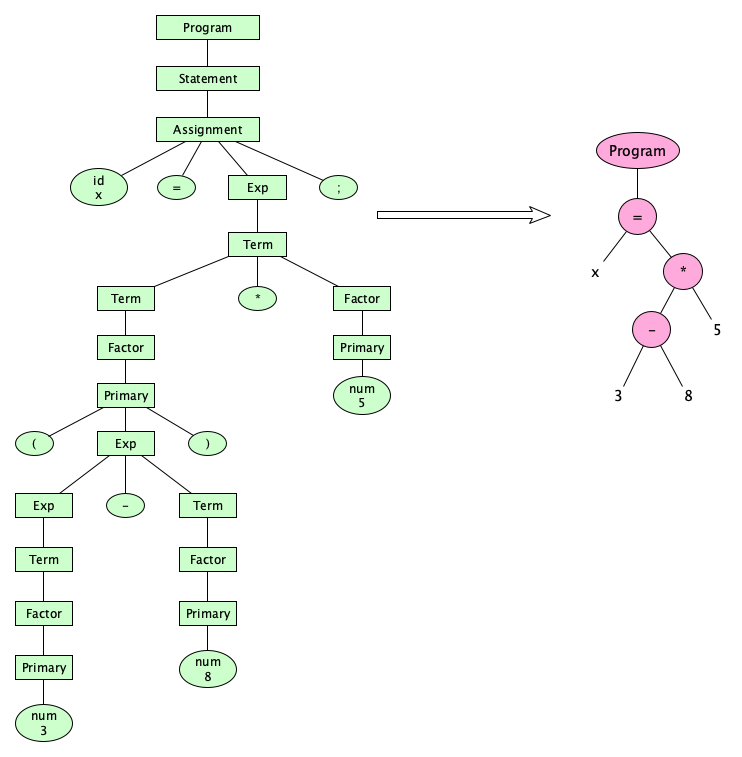So much simpler! And by the way, since these simpler trees have become known as abstract syntax trees (ASTs), it turns out that, yes, parse trees have become known as concrete syntax trees (CSTs).

How do we come up with an abstract syntax? Focus on what a program is trying to express, without worrying about punctuation. You don’t even have to worry about precedence and associativity (or even parentheses) because that stuff was already “taken care of” earlier. So cool! Here’s how we think about it for Astro:

• A program is a sequence of statements
• A statement is either an assignment or a call
• An assignment is an identifier being assigned an expression
• A call has an identifier for the function name and a list of expressions for its arguments
• An expression can be a number, identifier, a unary operator applied to an operand, two expressions with a binary operator, or a call.

If you want to formalize this, you can write a tree grammar:

$\begin{array}{lcl} n: \mathsf{Nml} & & \\ i: \mathsf{Ide} & & \\ e: \mathsf{Exp} & = & n \mid i \mid -e \mid e+e \mid e-e \mid e\,\mathtt{*}\,e \mid e\,/\,e \mid e\,\mathtt{\%}\,e \mid e\,\mathtt{**}\,e \mid i\;e*\\ s: \mathsf{Stm} & = & i = e \mid \mathtt{print}\;e\\ p: \mathsf{Pro} & = & \mathtt{program}\;s+\\ \end{array}$

Some people like to write tree grammars another way:

$\mathsf{Program} \rightarrow \mathsf{Stmt}+$
$\mathsf{Assign: Stmt} \rightarrow \mathsf{id}\;\mathsf{Exp}$
$\mathsf{Print: Stmt} \rightarrow \mathsf{Exp}$
$\mathsf{num: Exp}$
$\mathsf{id: Exp}$
$\mathsf{Negate: Exp} \rightarrow \mathsf{Exp}$
$\mathsf{Plus: Exp} \rightarrow \mathsf{Exp}\;\mathsf{Exp}$
$\mathsf{Minus: Exp} \rightarrow \mathsf{Exp}\;\mathsf{Exp}$
$\mathsf{Times: Exp} \rightarrow \mathsf{Exp}\;\mathsf{Exp}$
$\mathsf{Divide: Exp} \rightarrow \mathsf{Exp}\;\mathsf{Exp}$
$\mathsf{Reminder: Exp} \rightarrow \mathsf{Exp}\;\mathsf{Exp}$
$\mathsf{Power: Exp} \rightarrow \mathsf{Exp}\;\mathsf{Exp}$
$\mathsf{Call: Exp} \rightarrow \mathsf{id}\;\mathsf{Exp}*$

When translating to an abstract syntax, we not only get rid of intermediate syntax categories, but we also drop parentheses, commas, semicolons, brackets, and pretty much all punctuation. Some of the smaller keywords that you see in larger programming languages (in, to, of) also are not needed. Punctuation really only exists to give a “tree structure” to our linear program text. Once we have uncovered the tree structure in the CST, we just carry it over naturally to the AST.

Exercise: Give the token stream and draw the concrete and abstract syntax trees for the Astro program print(3-5-8-2*8-9-7**3**1);.
Exercise: Give the token stream and draw the concrete and abstract syntax trees for the Astro expression 98 * (247 - 6) / 3 / 100 - 701.

### Multiple Concrete Syntax Trees

Guess what else? ASTs is that they allow us to focus on programming language capabilities without worrying about syntactic details that people can reasonably have different preferences about. Take a look at this AST (in some hypothetical language, not Astro):CLASSWORK
Stop! Don’t scroll by! Study this tree! What is this program “saying”?

It appears to be a program in a language whose tree grammar probably looks like this:

$\begin{array}{lcl} n: \mathsf{Nml} & & \\ i: \mathsf{Ide} & & \\ t: \mathsf{Type} & = & \mathtt{int} \mid \mathtt{bool} \\ e: \mathsf{Exp} & = & n \mid i \mid -e \mid e+e \mid e-e \mid e\,\mathtt{*}\,e \mid e\,/\,e \mid e\,\mathtt{**}\,e \\ & & \mid\; e < e \mid e \leq e \mid e\mathtt\,{==}\,e \mid e \neq e \mid e > e \mid e \geq e \mid i\;e*\\ s: \mathsf{Stmt} & = & i = e \mid \mathtt{read}\;i* \mid \mathtt{write}\;e* \mid \mathtt{while}\;e\;b \\ d: \mathsf{Dec} & = & \mathtt{declare}\;(i\;t)*\\ b: \mathsf{Block} & = & {d*}\;s+\\ p: \mathsf{Program} & = & \mathtt{program}\;b\\ \end{array}$

But what does the actual concrete syntax look like? There’s no specific answer. There are an infinite number of possibilities! Here is just a small sampling of programs that could have generated that AST:

program:
var x, y: int
// In this language, indentation defines blocks
while y - 5 == 3:
var y: int
get(x)
get(y)
x = 2 * (3 + y)
put(5)


int x, y;
while y - 5 = 3 {
int y;
STDIN -> x;
STDIN -> y;
x <- 2 * (3 + y);
}
STDOUT <- 5;


COMMENT THIS LOOKS LIKE OLD CODE
DECLARE INT X.
DECLARE INT Y.
WHILE DIFFERENCE OF Y AND 5 IS 3 LOOP:
DECLARE INT Y.
MOVE PRODUCT OF 2 AND (SUM OF 3 AND Y) INTO X.
END LOOP.
WRITE 5 TO STDOUT.


(program
(declare x int)
(declare y int)
(while (= (- y 5) 3)
(define (y int))
(assign x (* 2 (+ 3 y)))
)
(write 5)
)


<program>
<declare var="x" type="int"/>
<declare var="y" type="int"/>
<while>
<equals>
<minus><varexp ref="y"/><intlit value="5"/></minus>
<intlit value="3"/>
</equals>
<declare var="y" type="int"/>
<assign varexp="x">
<times>
<intlit value="2"/>
<plus>
<intlit value="3"/>
<varexp ref="y"/>
</plus>
</times>
</assign>
</while>
<write>
<intlit value="5"/>
</write>
</program>

Exercise: Write the example in a JSON-like notation.
Exercise: The fourth example above has an “S-expression syntax” which is seen in languages like Lisp and Clojure. In such languages, concrete and abstract syntaxes can be said to be very close to each other. Why is this claim true?

### More AST Examples

Getting a good feel for abstract syntax naturally takes practice. Here are a couple examples to study to get a feel for how abstract we need to get. Here’s a fragment of Java:

if (((f(--x)))) {
System.out.println(- 3*q);
} else if (!here||there    &&everywhere) {
while (false) {
x += x >> 2 | 3  -++  x;
}
album.track("name") = title.scalars;
}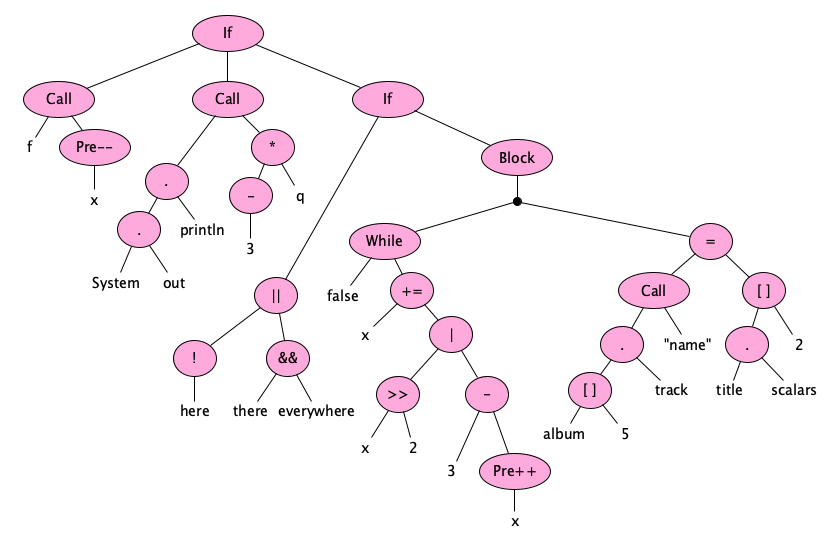And here’s a little JavaScript program:

export class C {
q() { const [p, q] = a.c}
r({x}, ...z) { while (c.c&&p|- -x) new C() }
}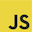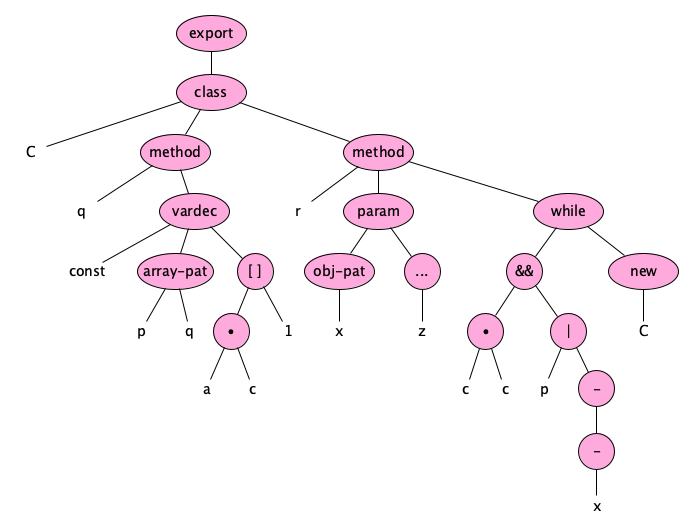## Syntax in the Real World

These notes were very much just an introduction to some of the theory surrounding syntax and syntax definitions. There is so much more to study. For example, you would do well to see how much work goes into writing a grammar for a real-world programming language. Here are a few to check out. Note the wide variety of notations:

Exercise: Browse each of these descriptions. Take notes that summarize the basic ideas of each. For each, how to they distinguish lexical from phrase syntax? How to they distinguish tokens from variables? What interesting conventions do they have that you had not thought of?

## Alternate Syntactic Descriptions

Hey, before we finish up, there’s one last thing to cover.

So far, we’ve seen two grammar notations, one generative (in previous notes on Language Theory) and one analytic (Ohm). The notation for generative grammars used here was cobbled together from a number of different established notations. It turns out there are dozens of published notations out there! You can use whatever you like, but there are some standardized forms that you should be aware of. The following four (CFG, BNF, EBNF, ABNF) are historically significant and very good to know. (You might even be expected to know these if you have a Computer Science degree.)

### Context-Free Grammars

Chomsky discovered (or invented?) his Type-2 grammars, which he called Context-Free Grammars, as a minimalistic formalism for studying languages that do not have context. They are by design very “mathematical” and far too low-level to be used in real life. They just use individual symbols. They have no special operators for alternation or repetition. They are too hard to read and are never used directly in practice. A CFG is a 4-tuple $(V,\Sigma,R,S)$, where $V$ is the set of variables, $\Sigma$ is the alphabet (of symbols in the language), $V \cap \Sigma = \varnothing$, $R \subseteq V \times (V \cup \Sigma)*$ is the rule set, and $S \in V$ is the start symbol. For the language of simple arithmetic expressions over natural numbers, a CFG is:

$\begin{array}{l}( \\ \;\;\; \{0, 1, 2, 3, 4, 5, 6, 7, 8, 9, +, -, *, /, (, )\}, \\ \;\;\; \{E, T, F, N, D\}, \\ \;\;\; \{ \\ \;\;\;\;\;\;\; (D, 0), (D, 1), (D, 2), (D, 3), (D, 4), (D, 5), (D, 6), \\ \;\;\;\;\;\;\; (D, 7), (D, 8), (D, 9), (N, D), (N, ND), (E, T), (E, E+T), \\ \;\;\;\;\;\;\; (E, E-T), (T,F), (T, T*F), (T, T/F), (F,N), (F, (E)) \\ \;\;\; \}, \\ \;\;\; E \\ )\end{array}$

Rather than writing them in tuple form, we often just list the rules, making sure the start symbol appears first, and making the variables and alphabet symbols inferrable by context:

E → E + T
E → E - T
E → T
T → T * F
T → T / F
T → F
F → ( E )
F → N
N → N D
N → D
D → 0
D → 1
D → 2
D → 3
D → 4
D → 5
D → 6
D → 7
D → 8
D → 9


A pure CFG is a super-minimal notation great for studying properties of languages. But when defining the syntax of real languages we want more expressiveness!

### BNF

With BNF, we add the ability to have our variables and alphabet symbols be written in a more readable form. To distinguish the two, we quote the variables with angle brackets. One special operator, | (meaning “or”), is permitted.

<expression> ::= <term>
|  <expression> + <term>
|  <expression> - <term>
<term>       ::= <factor>
|  <term> * <factor>
|  <term> / <factor>
<factor>     ::= <number>
|  ( <expression> )
<number>     ::= <digit> | <number> <digit>
<digit>      ::= 0 | 1 | 2 | 3 | 4 | 5 | 6 | 7 | 8 | 9


### EBNF

EBNF, or Extended Backus Naur Form, quotes the alphabet symbols instead of the variables, and adds operators for alternation (|), optionality (?), zero-or-more (*), and one-or-more (+).

EXP    = TERM (('+' | '-') TERM)*
TERM   = FACTOR (('*' | '/') FACTOR)*
FACTOR = NUMBER | '(' EXP ')'
NUMBER = ('0' | '1' | '2' | '3' | '4' | '5' | '6' | '7' | '8' | '9')+


Pure EBNF does not seem to have any mechanism for distinguishing lexical and phrase variables, so folks using EBNF will either write two grammars (one for the tokens, and one for the phrases), or use some other convention.

### ABNF

ABNF, or Augmented Backus Naur Form, is the specification used to describe all the Internet Protocols. It features tools for repetition, alternatives, order-independence, and value ranges.

exp    = term *(("+" / "-") term)
term   = factor *(("*" / "/") factor)
factor = number / "(" exp ")"
number = %x30-39


## Summary

We’ve covered:

• Motivation for Syntax
• Difference between syntax and semantics
• How to design a syntax
• Syntax diagrams
• Grammars
• Lexical vs. Phrase rules
• Ambiguity
• Precedence and associativity
• The problem of context
• Abstract syntax
• Tree grammars
• Syntax IRL
• Alternative syntax descriptions